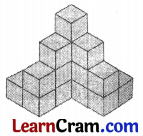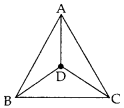# DAV Class 7 Maths Chapter 14 Worksheet 1 Solutions

The DAV Class 7 Maths Book Solutions and DAV Class 7 Maths Chapter 14 Worksheet 1 Solutions of Visualising Solids offer comprehensive answers to textbook questions.

## DAV Class 7 Maths Ch 14 WS 1 Solutions

Question 1.
Consider this pyramid ABODE (Fig.). Its base is a square BCDE. Find out how many more faces does it have? What is the shape of its other fares?Ii: has 4 more faces ABC, ACD, ADE, ABE, Shapes of other faces are triangular.

Question 2.
Name all the vertices, edges and faces of the given pyramid.
Vertices- A, B, C, D and E
Edges – AB, AC, AD, AE, CD, DE, EB and BC Faces – ABE, ABC, ACD, ADE and BEDCQuestion 3.
How many vertices, edges and faces will a cuboid have? What is the shape of its faces?Number of vertices = 8
Number of edges = 12
Number of faces = 6
Shape of faces = Rectangular

Question 4.
Consider the given figure. It shows a cube surmounted by a pyramid,(i) How many faces, edges and vertices does it have?
(ii) Name its edges, faces and vertices. Also state the faces which are triangles and which are squares.
(i) Number of faces = 9
Number of edges = 16
Number of vertices = 9

(ii) Name of edges are AB, BC, CD, AD, AE, EF, FG, GH, EH, CG, BF, DH, OA, OB, OC, OD.
Name of faces (a) Triangular AOB, BOC, COD, DOA (b) Square EFGH,
FGCB, CDHG, DHEA, ABFE.
Name of vertices: A, B, C, D, O, E, F, G, H### DAV Class 8 Maths Chapter 14 Worksheet 1 Notes

Two Dimensional figures:

The figures which can be drawn on a plane or surface are called two-dimensional figures or 2-D figures.
Example:3-Dimensional shapes:

The figures of solids which cannot be represented on a two-dimensional surface. However, their shapes can be drawn. 3-D solids can well be represented in space.
Example:• Solid shapes have vertices (corners), edges and faces (plane surfaces).
• A net is an outline (plane) which can be folded to make a solid. A solid can have more than one net.A solid can be sketched, in a plane in two forms:
(i) Oblique sketch
(ii) isometric sketch
In an isometric sketch, the dimensions tally with those of the solid.